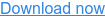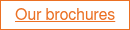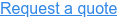# What is MPPT (Maximum Power Point Tracking)?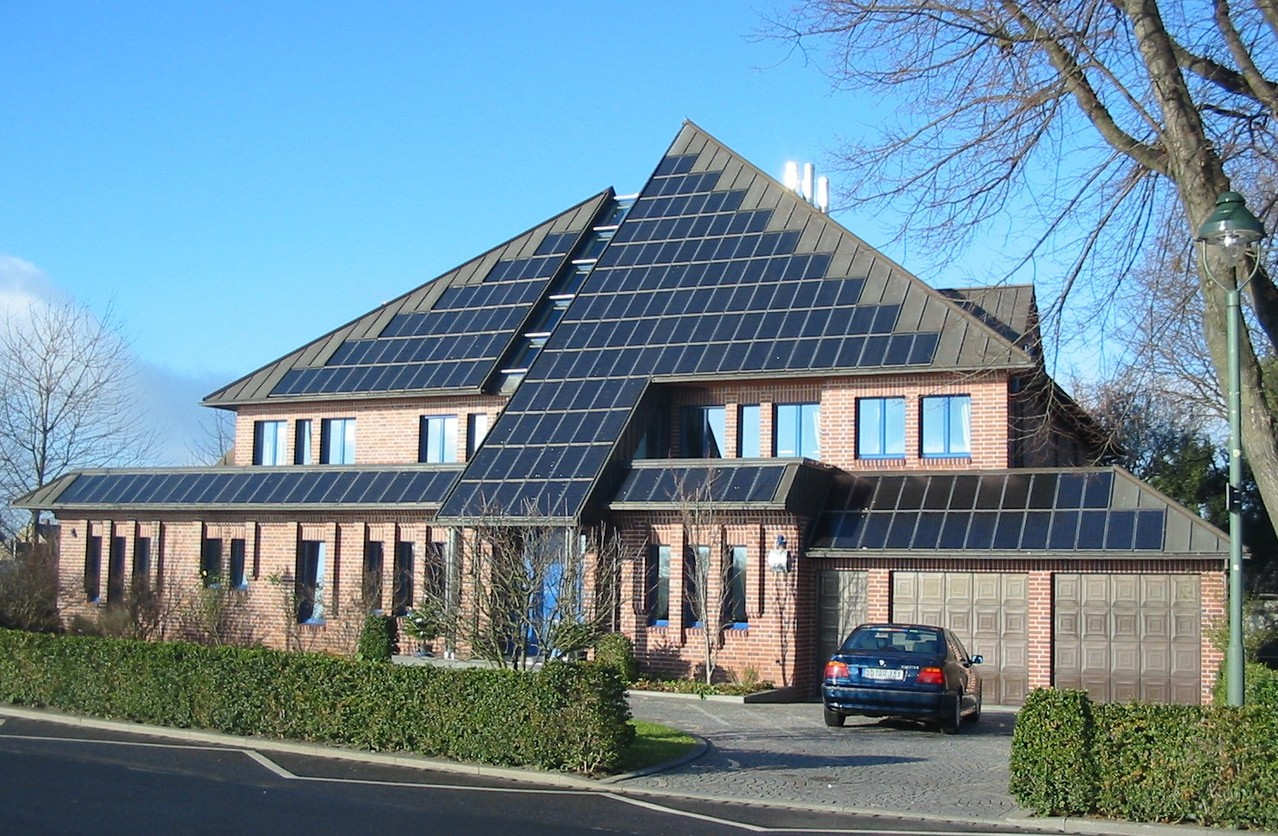### MPPT explained

To delve into Maximum Power Point Tracking (MPPT), as it relates to optimising the electronics of a solar PV system inverter, we need to start with an equation:

Power (W) = Volts (V) x Amps (A)

or, P = V x I

where P is the power (measured in Watts), I represents the current (measured in Amps) and V represents the Voltage (measured in Volts).

The concept of Maximum Power Point Tracking is probably best explained with reference to a sample Amps – Volts (I-V) curve, as follows:As the voltage across the panel varies, so does the current, in a manner similar to the curve above. The power generated by a solar panel is the product of volts x amps. There is a point on the curve at which power is maximised given by (Vmp, Imp), and therefore the load on the panel should be as close to Imp as possible.

Complexity arises because the I-V curve varies with the level of sunlight: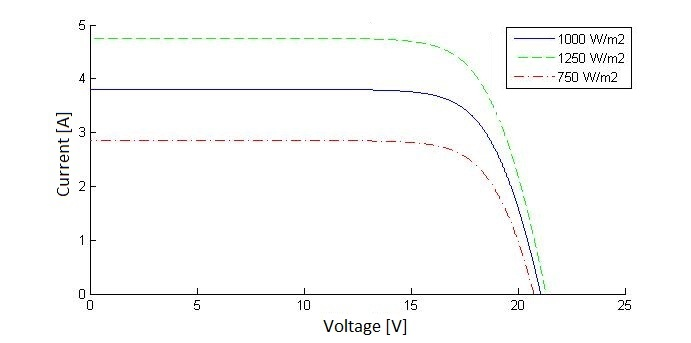As the level of radiation hitting the panel varies, the load current Imp and voltage Vmp at which power is maximised varies.

Maximum power point tracking (MPPT) is the process of continually finding this point and adjusting the load (resistance) to the panels such that power is maximised.

### String/central inverters: averaging equals compromise

With string inverters, Maximum Power Point Tracking is based on the ‘averaged’ I-V curve of a whole ‘string’ of panels (usually around 14). The inverter may allow for input of more than one string, in which case the MPPT for each individual string is independent of the other strings.

String-based MPPT is something of a compromise; the power produced will not be as high as if Maximum Power Point Tracking were to be applied at the individual panel level (as with micro-inverters and power optimisers), with the load applied to each panel optimised based on the environmental conditions (radiation, temperature) of that panel.

If some panels in a string are shaded and some are not, MPPT applied at the panel level will significantly boost the power output when compared to MPPT applied to a whole string of panels. If string inverters are being used, the design should take account of the likely differences in environmental conditions, stringing panels in similar environmental conditions (orientation, shading, temperature etc) together.

With central inverters, the averaging effect across ‘strings’ is more pronounced since the individual strings contain more panels.

Overall it is important to note that systems using micro-inverters or power optimisers will generate a higher output than systems using string inverters or central inverters.

### MPPT charge controllers

Maximum power point tracking charge controllers regulate the voltage and current going from the solar PV system to your home. They enable you to get the full rated power from your panels, rather than wasting a proportion of it as heat. Compared to a standard (non MPPT) charge controller, they provide 10-30% more power.